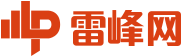图解 | NumPy可视化指南

2021/01/28 14:20NumPy是一个广泛适用的Python数据处理库，pandasOpenCV等库都基于numpy。同时，在PyTorchTensorFlowKeras等深度许欸小框架中，了解numpy将显著提高数据共享和处理能力，甚至无需过多更改就可以在GPU运行计算。

n维数组是NumPy的核心概念，这样的好处，尽管一维和而为数组的处理方式有些差异，但多数不同维数组的操作是一样的。本文将对以下三个部分展开介绍：

1. 向量——一维数组

2. 矩阵——二维数组

3. 3维及更高维数组

numpy数组 vs. Python列表• 更紧凑，高维时尤为明显

• 向量化后运算速度比列表更快

• 在末尾添加元素时不如列表高效

• 元素类型一般比较固定1.向量与1维数组

向量初始化arange浮点类型数据不是非常友好：向量索引anyall与其他Python使用类似向量操作

NumPy的计算速度是其亮点之一，其向量运算操作接近C++级别，避免了Python循环耗时较多的问题。NumPy允许像普通数字一样操作整个数组：numpy提供了许多数学函数来处理矢量：numpy也提供了如下三角函数运算：floor向上取整，ceil向下取整，round四舍五入

np.around与np.round是等效的，这样做只是为了避免 from numpy import *时与Python  aroun的冲突（但一般的使用方式是import numpy as np）。当然，你也可以使用a.round()。

numpy还可以实现以下功能：查找向量中的元素index()中的方括号表示j或i&j可以省略

• 可以通过np.where(a==x) 查找元素，但这种方法很不pythonic，哪怕需要查找的项在数组开头，该方法也需要遍历整个数组。

• 使用Numba实现加速查找，next((i for i, v in np.ndenumerate(a) if v==x), -1)，在最坏的情况下，它的速度要比where慢。

• 如果数组是排好序的，使用v = np.searchsorted(a, x); return v if a[v]==x else -1时间复杂度为O(log N)，但在这之前，排序的时间复杂度为O(N log N)。

浮点数比较

np.allclose(a, b)用于容忍误差之内的浮点数比较。• np.allclose假定所有比较数字的尺度为1。如果在纳秒级别上，则需要将默认atol参数除以1e9：np.allclose(1e-9,2e-9, atol=1e-17)==False。

• math.isclose不对要比较的数字做任何假设，而是需要用户提供一个合理的abs_tol值（np.allclose默认的atol值1e-8足以满足小数位数为1的浮点数比较，即math.isclose(0.1+0.2–0.3, abs_tol=1e-8)==True。

2.矩阵和二维数组“view”表示数组切片时并未进行任何复制，在修改数组后，相应更改也将反映在切片中。

轴参数矩阵运算行向量与列向量矩阵操作delete可以删除特定的行或列：Meshgridsmeshgrid函数接受任意一组索引，通过mgrid切片和indices索引生成完整的索引范围，然后，fromfunction函数根据I和J实现运算。矩阵统计np.amin等同于np.min，这样做同样是为了避免from numpy import *可能的歧义。

2维及更高维中的argmin和argmax函数分别返回最小和最大值的索引，通过unravel_index函数可以将其转换为二维坐标：allany同样也可作用于特定维度：矩阵排序1、a[a [:,0] .argsort()]表示按第一列对数组进行排序：a = a[a[:,2].argsort()]

a = a[a[:,1].argsort(kind='stable')]

a = a[a[:,0].argsort(kind='stable')]2、函数lexsort可以像上述这样对所有列进行排序，但是它总是按行执行，并且排序的行是颠倒的（即从下到上），其用法如下：

a[np.lexsort(np.flipud(a[2,5].T))]，首先按第2列排序，然后按第5列排序；a[np.lexsort(np.flipud(a.T))]，从左到右依次排序各列。3、sort函数还有一个order参数，但该方法极不友好，不推荐学习。

4、在pandas中排序也是不错的选择，因为在pandas中操作位置确定，可读性好且不易出错：

- pd.DataFrame(a).sort_values(by=[2,5]).to_numpy()，先按第2列排序，再按第5列排序。

-pd.DataFrame(a).sort_values().to_numpy()，按从左到右的顺序对所有列进行排序。

3、3维及更高维数组参考

1. Scott Sievert, NumPy GPU acceleration

2. Jay Alammar, A Visual Intro to NumPy and Data Representation

3. Big-O Cheat Sheet site

4. Python Time Complexity wiki page

5. NumPy Issue #14989, Reverse param in ordering functions

6. NumPy Issue #2269, First nonzero element

7. Numba library homepage

8. The Floating-Point Guide, Comparison

9. NumPy Issue #10161, numpy.isclose vs math.isclose

10. 100 NumPy exercises on GitHub

AI研习社是AI学术青年和AI开发者技术交流的在线社区。我们与高校、学术机构和产业界合作，通过提供学习、实战和求职服务，为AI学术青年和开发者的交流互助和职业发展打造一站式平台，致力成为中国最大的科技创新人才聚集地。图解 | NumPy可视化指南